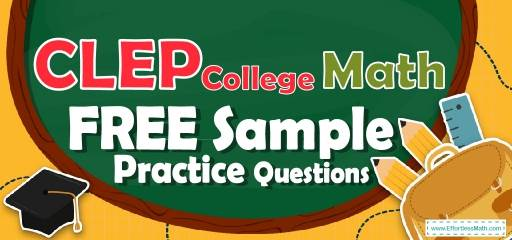# CLEP College Math FREE Sample Practice QuestionsPreparing for the CLEP College Math test? To do your best on the CLEP College Math test, you need to review and practice real CLEP College Math questions.  There’s nothing like working on CLEP College Math sample questions to hone your math skills and put you more at ease when taking the CLEP College Math test. The sample math questions you’ll find here are brief samples designed to give you the insights you need to be as prepared as possible for your CLEP College Math test.

Check out our sample CLEP College Math practice questions to find out what areas you need to practice more before taking the CLEP College Math test!

Start preparing for the 2022 CLEP College Math test with our free sample practice questions. Also, make sure to follow some of the related links at the bottom of this post to get a better idea of what kind of mathematics questions you need to practice.

## 10 Sample CLEP College Math Practice Questions

1- A bag contains $$18$$ balls: two green, five black, eight blue, a brown, a red, and one white. If $$17$$ balls are removed from the bag at random, what is the probability that a brown ball has been removed?

A. $$\frac{1}{9}$$

B. $$\frac{1}{6}$$

C. $$\frac{16}{18}$$

D. $$\frac{17}{18}$$

2- The average of five consecutive numbers is $$36$$. What is the smallest number?

A. 38

B. 36

C. 34

D. 12

3- The price of a car was $$\28,000$$ in $$2012$$. In $$2013$$, the price of that car was $$\18,200$$. What was the rate of depreciation of the price of a car per year?

A. $$20\%$$

B. $$30\%$$

C. $$35\%$$

D. $$40\%$$

4- The width of a box is one-third of its length. The height of the box is one-third of its width. If the length of the box is $$36$$ cm, what is the volume of the box?

A. $$81 cm^3$$

B. $$162 cm^3$$

C. $$243 cm^3$$

D. $$1,728 cm^3$$

5- A tree $$32$$ feet tall casts a shadow $$12$$ feet long. Jack is $$6$$ feet tall. How long is Jack’s shadow?

A. $$2.25$$ feet

B. $$4$$ feet

C. $$4.25$$ feet

D. $$8$$ feet

6- When a number is subtracted from $$28$$ and the difference is divided by that number, the result is $$3$$. What is the value of the number?

A. 2

B. 4

C. 7

D. 12

7- An angle is equal to the one-ninth of its supplement. What is the measure of that angle?

A. 9

B. 18

C. 25

D. 60

8- John traveled $$150$$ km in $$6$$ hours and Alice traveled $$140$$ km in $$4$$ hours. What is the ratio of the average speed of John to the average speed of Alice?

A. $$3∶2$$

B. $$2∶3$$

C. $$5∶7$$

D. $$5∶6$$

9- If $$A={2,5,11,15}, B={1,2,3,4,5,6}$$, and $$C={5,7,9,11,13}$$, then which of the following set is $$(A∪B)∩C$$?

A. $${1,2,3,4,5,6,11,15}$$

B. $${1,2,3,4,5,6,7,11,13,15}$$

C. $${5,11,13,15}$$

D. $${5,11}$$

10- If $$4n-3≥1$$, what is the least possible value of $$4n+3$$?

A. 3

B. 4

C. 7

D. 9

## Best CLEP College Math Prep Resource for 2022

1- D
If $$17$$ balls are removed from the bag at random, there will be one ball in the bag. The probability of choosing a brown ball is $$1$$ out of $$18$$. Therefore, the probability of not choosing a brown ball is $$17$$ out of $$18$$ and the probability of having not a brown ball after removing $$17$$ balls is the same.

2- C
Let $$x$$ be the smallest number. Then, these are the numbers: $$x, x+1, x+2, x+3, x+4$$
average$$=\frac{sum \ of \ terms }{number \ of \ terms}⇒36=\frac{x+(x+1)+(x+2)+(x+3)+(x+4)}{5}⇒36=\frac{5x+10}{5 }⇒$$
$$180=5x+10 ⇒170= 5x⇒x=34$$

3- C
Use this formula: Percent of Change: $$\frac{New \ Value-Old \ Value}{Old \ Value}×100\%$$ .
$$\frac{18,200-28,000}{28,000}×100\%=-35\%$$. The negative sign means that the price decreased

4- D
If the length of the box is $$36$$, then the width of the box is one-third of it, $$12$$, and the height of the box is $$4$$ (one-third of the width). The volume of the box is: V=lwh=$$(36)(12)(4)=1,728$$

5- A
Write a proportion and solve for the missing number. $$\frac{32}{12}=\frac{6}{x}→32x=6×12=72$$
$$32x=72→x=\frac{72}{32}=2.25$$

6- C
Let $$x$$ be the number. Write the equation and solve for $$x$$. $$(28-x)÷x= 3$$
Multiply both sides by $$x. (28-x)= 3x$$, then add $$x$$ both sides. $$28=4x$$, now divide both sides by $$4. x=7$$

7- B
The sum of supplement angles is $$180$$. Let $$x$$ be that angle. Therefore, $$x+9x=180$$
$$10x=180$$, divide both sides by $$10: x=18$$

8- C
The average speed of john is: $$150÷ 6=25$$, The average speed of Alice is: $$140÷4=35$$
Write the ratio and simplify. $$25: 35 ⇒ 5∶7$$

9- D
The union of A and B is: $$A∪B={1,2,3,4,5,6,11,15}$$
The intersection of $$(A∪B)$$ and C is: $$(A∪B)∩C={5,11}$$

10- C
Adding $$6$$ to each side of the inequality $$4n – 3 ≥ 1$$ yields the inequality $$4n + 3 ≥ 7$$. Therefore, the least possible value of $$4n + 3$$ is $$7$$.

Looking for the best resource to help you succeed on the CLEP College Math test?

## The Best Books to Ace the CLEP College Math Test

### What people say about "CLEP College Math FREE Sample Practice Questions - Effortless Math: We Help Students Learn to LOVE Mathematics"?

No one replied yet.

X
52% OFF

Limited time only!

Save Over 52%

SAVE $40 It was$76.99 now it is \$36.99